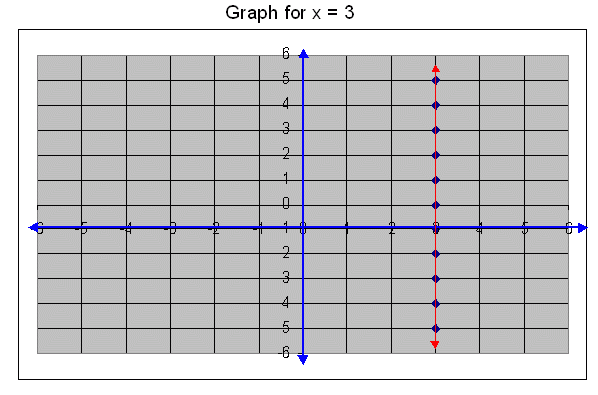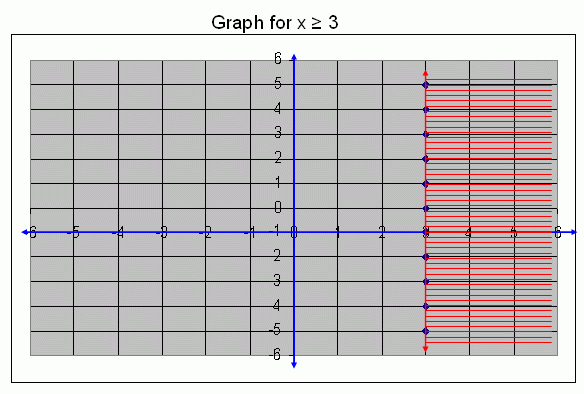Email us to get an instant 20% discount on highly effective K-12 Math & English kwizNET Programs!

#### Online Quiz (WorksheetABCD)

Questions Per Quiz = 2 4 6 8 10

### Middle/High School Algebra, Geometry, and Statistics (AGS)2.6 Graph for x ł 3

 Example: Draw a graph for x ł 3. Solution: First we draw a graph for a straight line x = 3. It means that in this straight line the x coordinate is constant that is 3.x ł 3 means, that x = 3 or x > 3, all these points are satisfies this condition. Therefore, the graph for x ł 3 is,Directions: Draw the graphs for the following condition. 1. Draw a graph for x ł 3. 2. Draw a graph for x ł 2. 3. Draw a graph for x ł 6. 4. Draw a graph for x ł 4. 5. Draw a graph for x ł 1.

#### Subscription to kwizNET Learning System offers the following benefits:

• Unrestricted access to grade appropriate lessons, quizzes, & printable worksheets
• Instant scoring of online quizzes
• Progress tracking and award certificates to keep your student motivated
• Unlimited practice with auto-generated 'WIZ MATH' quizzes